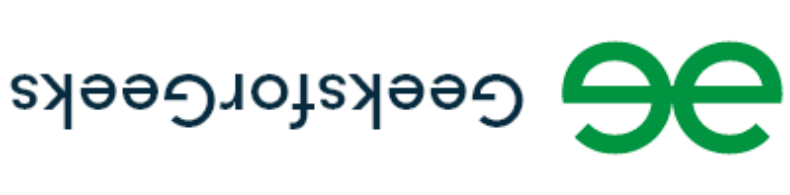GeeksforGeeks App
Open AppBrowser
Continue

# Image Processing in Java – Changing Orientation of Image

Prerequisites:

### Changing the Orientation of Image

Here we will use OpenCV to change the orientation of any input image, by using the CORE.flip() method of the OpenCV library. The main idea is that an input buffered image object will be converted to a mat object and then a new mat object will be created in which the original mat object values are put after orientation modification. For achieving the above result, we will be requiring some of the OpenCV methods:

Implementation: Let the sample image be as follows:Example:

## Java

 `// Java program to Illustrate How to Change``// Orientation of an Image`` ` `// Importing required classes``import` `java.awt.image.BufferedImage;``import` `java.awt.image.DataBufferByte;``import` `java.io.File;``import` `java.io.IOException;``import` `javax.imageio.ImageIO;``import` `org.opencv.core.Core;``import` `org.opencv.core.CvType;``import` `org.opencv.core.Mat;`` ` `// Main class``// OrientingImage``public` `class` `GFG {`` ` `    ``// Main driver method``    ``public` `static` `void` `main(String[] args)``        ``throws` `IOException``    ``{`` ` `        ``// Loading methods of the opencv library``        ``System.loadLibrary(Core.NATIVE_LIBRARY_NAME);`` ` `        ``// Passing the input image as path from local``        ``// directory by creating object of File class``        ``File input = ``new` `File(``"E:\\test.jpg"``);`` ` `        ``// Reading image``        ``BufferedImage image = ImageIO.read(input);`` ` `        ``// Converting buffered image object to mat object``        ``byte``[] data = ((DataBufferByte)image.getRaster()``                           ``.getDataBuffer())``                          ``.getData();``        ``Mat mat = ``new` `Mat(image.getHeight(),``                          ``image.getWidth(), CvType.CV_8UC3);``        ``mat.put(``0``, ``0``, data);`` ` `        ``// Creating a new mat object and``        ``// putting the modified input mat object``        ``// using flip() method``        ``Mat newMat``            ``= ``new` `Mat(image.getHeight(), image.getWidth(),``                      ``CvType.CV_8UC3);`` ` `        ``// Fipping the image about both axis``        ``Core.flip(mat, newMat, -``1``);`` ` `        ``// Converting the newly created mat object to``        ``// buffered image object``        ``byte``[] newData``            ``= ``new` `byte``[newMat.rows() * newMat.cols()``                       ``* (``int``)(newMat.elemSize())];``        ``newMat.get(``0``, ``0``, newData);``        ``BufferedImage image1 = ``new` `BufferedImage(``            ``newMat.cols(), newMat.rows(), ``5``);``        ``image1.getRaster().setDataElements(``            ``0``, ``0``, newMat.cols(), newMat.rows(), newData);`` ` `        ``// Storing the output image by``        ``// creating object of File class``        ``File output = ``new` `File(``"E:\\result.jpg"``);``        ``ImageIO.write(image1, ``"jpg"``, output);``    ``}``}`

Output:Note: Do not scale for resolution as the output image is appended bigger to perceive better, it will be generated on IDE of same size as that of resolution and size passed of the input image.